# Maharashtra Board Class 12 Physics Solutions Chapter 1 Rotational Dynamics

Balbharti Maharashtra State Board 12th Physics Textbook Solutions Chapter 1 Rotational Dynamics Textbook Exercise Questions and Answers.

## Maharashtra State Board 12th Physics Solutions Chapter 1 Rotational Dynamics

1. Choose the correct option.

i) When seen from below, the blades of a ceiling fan are seen to be revolving anticlockwise and their speed is decreasing. Select the correct statement about the directions of its angular velocity and angular acceleration.
(A) Angular velocity upwards, angular acceleration downwards.
(B) Angular velocity downwards, angular acceleration upwards.
(C) Both, angular velocity and angular acceleration, upwards.
(D) Both, angular velocity and angular acceleration, downwards.
(A) Angular velocity upwards, angular acceleration downwards.

ii) A particle of mass 1 kg, tied to a 1.2 m long string is whirled to perform vertical circular motion, under gravity. Minimum speed of a particle is 5 m/s. Consider the following statements.
P) Maximum speed must be 5 5 m/s.
Q) Difference between maximum and minimum tensions along the string is 60 N. Select correct option.
(A) Only statement P is correct.
(B) Only statement Q is correct.
(C) Both the statements are correct.
(D) Both the statements are incorrect.
(C) Both the statements are correct.iii) Select correct statement about the formula (expression) of moment of inertia (M.I.) in terms of mass M of the object and some of its distance parameter/s, such as R, L, etc.
(A) Different objects must have different expressions for their M.I.
(B) When rotating about their central axis, a hollow right circular cone and a disc have the same expression for the M.I.
(C) Expression for the M.I. for a parallelepiped rotating about the transverse axis passing through its centre includes its depth.
(D) Expression for M.I. of a rod and that of a plane sheet is the same about a transverse axis.
(B) When rotating about their central axis, a hollow right circular cone and a disc have the same expression for the M.I.

iv) In a certain unit, the radius of gyration of a uniform disc about its central and transverse axis is $$\sqrt{2.5}$$. Its radius of gyration about a tangent in its plane (in the same unit) must be
(A) $$\sqrt{5}$$
(B) 2.5
(C) 2$$\sqrt{2.5}$$
(D) $$\sqrt{12.5}$$
(B) 2.5

v) Consider following cases:
(P) A planet revolving in an elliptical orbit.
(Q) A planet revolving in a circular orbit.
Principle of conservation of angular momentum comes in force in which of these?
(A) Only for (P)
(B) Only for (Q)
(C) For both, (P) and (Q)
(D) Neither for (P), nor for (Q)
(C) For both, (P) and (Q)

X) A thin walled hollow cylinder is rolling down an incline, without slipping. At any instant, the ratio ”Rotational K.E.:
Translational K.E.: Total K.E.” is
(A) 1:1:2
(B) 1:2:3
(C) 1:1:1
(D) 2:1:3
(D) 2:1:3

i) Why are curved roads banked?
A car while taking a turn performs circular motion. If the road is level (or horizontal road), the necessary centripetal force is the force of static friction between the car tyres and the road surface. The friction depends upon the nature of the surfaces in contact and the presence of oil and water on the road. If the friction is inadequate, a speeding car may skid off the road. Since the friction changes with circumstances, it cannot be relied upon to provide the necessary centripetal force. Moreover, friction results in fast wear and tear of the tyres.

To avoid the risk of skidding as well as to reduce the wear and tear of the car tyres, the road surface at a bend is tilted inward, i.e., the outer side of the road is raised above its inner side. This is called banking of road. On a banked road, the resultant of the normal reaction and the gravitational force can act as the necessary centripetal force. Thus, every car can be safely driven on such a banked curve at certain optimum speed, without depending on friction. Hence, a road should be properly banked at a bend.

The angle of banking is the angle of inclination of the surface of a banked road at a bend with the horizontal.

ii) Do we need a banked road for a two wheeler? Explain.
When a two-wheeler takes a turn along an unbanked road, the force of friction provides the centripetal force. The two-wheeler leans inward to counteract a torque that tends to topple it outward. Firstly, friction cannot be relied upon to provide the necessary centripetal force on all road conditions. Secondly, the friction results in wear and tear of the tyres. On a banked road at a turn, any vehicle can negotiate the turn without depending on friction and without straining the tyres.

iii) On what factors does the frequency of a conical pendulum depend? Is it independent of some factors?
The frequency of a conical pendulum, of string length L and semivertical angle θ, is
n = $$\frac{1}{2 \pi} \sqrt{\frac{g}{L \cos \theta}}$$
where g is the acceleration due to gravity at the place.
From the above expression, we can see that

1. n ∝ $$\sqrt{g}$$
2. n ∝ $$\frac{1}{\sqrt{L}}$$
3. n ∝ $$\frac{1}{\sqrt{\cos \theta}}$$
(if θ increases, cos θ decreases and n increases)
4. The frequency is independent of the mass of the bob.

iv) Why is it useful to define radius of gyration?
Definition : The radius of gyration of a body rotating about an axis is defined as the distance between the axis of rotation and the point at which the entire mass of the body can be supposed to be concentrated so as to give the same moment of inertia as that of the body about the given axis.The moment of inertia (MI) of a body about a given rotation axis depends upon

1. the mass of the body and
2. the distribution of mass about the axis of rotation. These two factors can be separated by expressing the MI as the product of the mass (M) and the square of a particular distance (k) from the axis of rotation. This distance is called the radius of gyration and is defined as given above. Thus,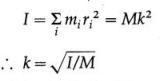Physical significance : The radius of gyration is less if I is less, i.e., if the mass is distributed close to the axis; and it is more if I is more, i.e., if the mass is distributed away from the axis. Thus, it gives the idea about the distribution of mass about the axis of rotation.

v) A uniform disc and a hollow right circular cone have the same formula for their M.I., when rotating about their central axes. Why is it so?
The radius of gyration of a thin ring of radius Rr about its transverse symmetry axis is
Kr = $$\sqrt{I_{\mathrm{CM}} / M_{\mathrm{r}}}$$ = $$\sqrt{R_{\mathrm{r}}^{2}}$$ = Rr
The radius of gyration of a thin disc of radius Rd about its transverse symmetry axis is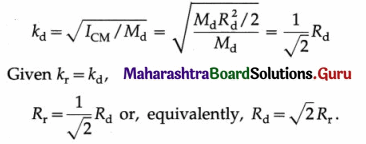Question 3.
While driving along an unbanked circular road, a two-wheeler rider has to lean with the vertical. Why is it so? With what angle the rider has to lean? Derive the relevant expression. Why such a leaning is not necessary for a four wheeler?
When a bicyclist takes a turn along an unbanked road, the force of friction $$\vec{f}_{\mathrm{s}}$$ provides the centripetal force; the normal reaction of the road $$\vec{N}$$ is vertically up. If the bicyclist does not lean inward, there will be an unbalanced outward torque about the centre of gravity, fs.h, due to the friction force that will topple the bicyclist outward. The bicyclist must lean inward to counteract this torque (and not to generate a centripetal force) such that the opposite inward torque of the couple formed by $$\vec{N}$$ and the weight $$\vec{g}$$, mg.a = fs.h1Since the force of friction provides the centripetal force,
fs = $$\frac{m v^{2}}{r}$$
If the cyclist leans from the vertical by an angle 9, the angle between $$\vec{N}$$ and $$\vec{F}$$ in above figure.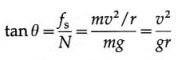Hence, the cyclist must lean by an angle
θ = tan-1$$\left(\frac{v^{2}}{g r}\right)$$

When a car takes a turn along a level road, apart from the risk of skidding off outward, it also has a tendency to roll outward due to an outward torque about the centre of gravity due to the friction force. But a car is an extended object with four wheels. So, when the inner wheels just get lifted above the ground, it can be counterbalanced by a restoring torque of the couple formed by the normal reaction (on the outer wheels) and the weight.

Question 4.
Using the energy conservation, derive the expressions for the minimum speeds at different locations along a vertical circular motion controlled by gravity. Is zero speed possible at the uppermost point? Under what condition/s? Also prove that the difference between the extreme tensions (or normal forces) depends only upon the weight of the object.
In a non uniform vertical circular motion, e.g., those of a small body attached to a string or the loop-the-loop manoeuvers of an aircraft or motorcycle or skateboard, the body must have some minimum speed to reach the top and complete the circle. In this case, the motion is controlled only by gravity and zero speed at the top is not possible.

However, in a controlled vertical circular motion, e.g., those of a small body attached to a rod or the giant wheel (Ferris wheel) ride, the body or the passenger seat can have zero speed at the top, i.e., the motion can be brought to a stop.

Question 5.
Discuss the necessity of radius of gyration. Define it. On what factors does it depend and it does not depend? Can you locate some similarity between the centre of mass and radius of gyration? What can you infer if a uniform ring and a uniform disc have the same radius of gyration?
Definition : The radius of gyration of a body rotating about an axis is defined as the distance between the axis of rotation and the point at which the entire mass of the body can be supposed to be concentrated so as to give the same moment of inertia as that of the body about the given axis.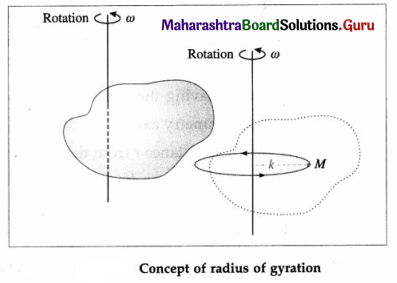The moment of inertia (MI) of a body about a given rotation axis depends upon

1. the mass of the body and
2. the distribution of mass about the axis of rotation. These two factors can be separated by expressing the MI as the product of the mass (M) and the square of a particular distance (k) from the axis of rotation. This distance is called the radius of gyration and is defined as given above. Thus,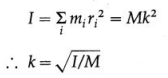Physical significance : The radius of gyration is less if I is less, i.e., if the mass is distributed close to the axis; and it is more if I is more, i.e., if the mass is distributed away from the axis. Thus, it gives the idea about the distribution of mass about the axis of rotation.

The centre of mass (CM) coordinates locates a point where if the entire mass M of a system of particles or that of a rigid body can be thought to be concentrated such that the acceleration of this point mass obeys Newton’s second law of motion, viz.,
$$\vec{F}_{\mathrm{net}}$$ = M$$\overrightarrow{\mathrm{a}}_{\mathrm{CM}}$$, where $$\vec{F}_{\mathrm{net}}$$ is the sum of all the external forces acting on the body or on the individual particles of the system of particles.

Similarly, radius of gyration locates a point from the axis of rotation where the entire mass M can be thought to be concentrated such that the angular acceleration of that point mass about the axis of rotation obeys the relation, $$\vec{\tau}_{\mathrm{net}}$$ = M$$\vec{\alpha}$$, where $$\vec{\tau}_{\text {net }}$$ is the sum of all the external torques acting on the body or on the individual particles of the system of particles.Question 6.
State the conditions under which the theorems of parallel axes and perpendicular axes are applicable. State the respective mathematical expressions.
The theorem of parallel axis is applicable to any body of arbitrary shape. The moment of inertia (MI) of the body about an axis through the centre mass should be known, say, ICM. Then, the theorem can be used to find the MI, I, of the body about an axis parallel to the above axis. If the distance between the two axes is h,
I = ICM + Mh2 …(1)
The theorem of perpendicular axes is applicable to a plane lamina only. The moment of inertia Iz of a plane lamina about an axis-the z axis- perpendicular to its plane is equal to the sum of its moments of inertia Ix and Iy about two mutually perpendicular axes x and y in its plane and through the point of intersection of the perpendicular axis and the lamina.
Iz = Ix + Iy …. (2)

Question 7.
Derive an expression that relates angular momentum with the angular velocity of a rigid body.
Consider a rigid body rotating with a constant angular velocity $$\vec{\omega}$$ about an axis through the point O and perpendicular to the plane of the figure. All the particles of the body perform uniform circular motion about the axis of rotation with the same angular velocity $$\vec{\omega}$$. Suppose that the body consists of N particles of masses m1, m2, …, mn, situated at perpendicular distances r1, r2, …, rN, respectively from the axis of rotation.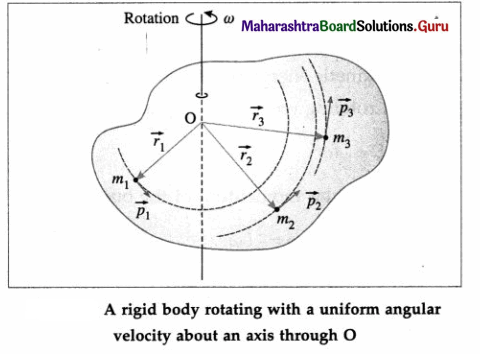The particle of mass m1 revolves along a circle of radius r1, with a linear velocity of magnitude v1 = r1ω. The magnitude of the linear momentum of the particle is
p1 = m1v1 = m1r1ω
The angular momentum of the particle about the axis of rotation is by definition,
$$\vec{L}_{1}$$ = $$\vec{r}_{1}$$ × $$\vec{p}_{1}$$
∴ L1 = r1p1 sin θ
where θ is the smaller of the two angles between $$\vec{r}_{1}$$ and $$\vec{p}_{1} \text { . }$$
In this case, θ = 90° ∴ sin θ = 1
∴ L1 = r1p1 = r1m1r1ω = m1r12ω
Similarly L2 = m2r22ω, L3 = m3r32ω, etc.
The angular momentum of the body about the given axis is
L = L1 + L2 + … + LN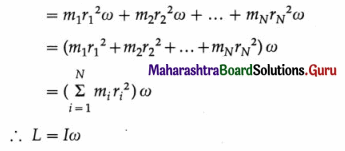where I = $$\sum_{i=1}^{N} m_{i} r_{i}^{2}$$ = moment of inertia of the body about the given axis.
In vector form, $$\vec{L}$$ = $$I \vec{\omega}$$
Thus, angular momentum = moment of inertia × angular velocity.
[Note : Angular momentum is a vector quantity. It has the same direction as $$\vec{\omega}$$.]

Question 8.
Obtain an expression relating the torque with angular acceleration for a rigid body.
A torque acting on a body produces angular acceleration. Consider a rigid body rotating about an axis passing through the point O and perpendicular to the plane of the figure. Suppose that a torque $$\vec{\tau}$$ on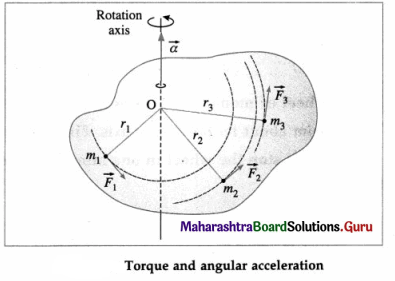the body produces uniform angular acceleration $$\vec{\alpha}$$ along the axis of rotation.
The body can be considered as made up of N particles with masses m1, m2, …, mN situated at perpendicular distances r1, r2, …, rN respectively from the axis of rotation, $$\vec{\alpha}$$ is the same for all the particles as the body is rigid. Let $$\vec{F}_{1}$$, $$\vec{F}_{2}$$, …, $$\vec{F}_{N}$$ be the external forces on the particles.
The torque $$\vec{\tau}_{1}$$, on the particle of mass m1, is
$$\vec{\tau}_{1}$$ = $$\vec{r}_{1}$$ × $$\vec{F}_{1}$$
∴ τ1 = r1F1 sin θ
where θ is the smaller of the two angles between $$\vec{r}_{1}$$ and $$\vec{F}_{1} .$$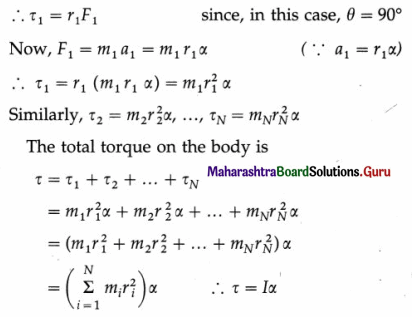whereis the moment of inertia of the body about the axis of rotation.
In vector form, $$\vec{\tau}$$ = I$$\vec{\alpha}$$
This gives the required relation.
Angular acceleration $$\vec{\alpha}$$ has the same direction as the torque $$\vec{\tau}$$ and both of them are axial vectors along the rotation axis.

Question 9.
State and explain the principle of conservation of angular momentum. Use a suitable illustration. Do we use it in our daily life? When?
Law (or principle) of conservation of angular momentum : The angular momentum of a body is conserved if the resultant external torque on the body is zero.
Explanation : This law (or principle) is used by a figure skater or a ballerina to increase their speed of rotation for a spin by reducing the body’s moment of inertia. A diver too uses it during a somersault for the same reason.

(1) Ice dance :
Twizzle and spin are elements of the sport of figure skating. In a twizzle a skater turns several revolutions while travelling on the ice. In a dance spin, the skater rotates on the ice skate and centred on a single point on the ice. The torque due to friction between the ice skate and the ice is small. Consequently, the angular momentum of a figure skater remains nearly constant.

For a twizzle of smaller radius, a figure skater draws her limbs close to her body to reduce moment of inertia and increase frequency of rotation. For larger rounds, she stretches out her limbs to increase moment of inertia which reduces the angular and linear speeds.

A figure skater usually starts a dance spin in a crouch, rotating on one skate with the other leg and both arms extended. She rotates relatively slowly because her moment of inertia is large. She then slowly stands up, pulling the extended leg and arms to her body. As she does so, her moment of inertia about the axis of rotation decreases considerably,and thereby her angular velocity substantially increases to conserve angular momentum.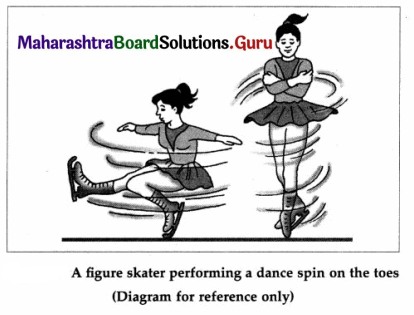(2) Diving :
Take-off from a springboard or diving platform determines the diver’s trajectory and the magnitude of angular momentum. A diver must generate angular momentum at take-off by moving the position of the arms and by a slight hollowing of the back. This allows the diver to change angular speeds for twists and somersaults in flight by controlling her/his moment of inertia. A compact tucked shape of the body lowers the moment of inertia for rotation of smaller radius and increased angular speed. The opening of the body for the vertical entry into water does not stop the rotation, but merely slows it down. The angular momentum remains constant throughout the flight.Question 10.
Discuss the interlink between translational, rotational and total kinetic energies of a rigid object that rolls without slipping.
Consider a symmetric rigid body, like a sphere or a wheel or a disc, rolling on a plane surface with friction along a straight path. Its centre of mass (CM) moves in a straight line and, if the frictional force on the body is large enough, the body rolls without slipping. Thus, the rolling motion of the body can be treated as translation of the CM and rotation about an axis through the CM. Hence, the kinetic energy of a rolling body is
E = Etran + Erot ……. (1)

where Etran and Erot are the kinetic energies associated with translation of the CM and rotation about an axis through the CM, respectively.

Let M and R be the mass and radius of the body. Let ω, k and i be the angular speed, radius of gyration and moment of inertia for rotation about an axis through its centre, and v be the translational speed of the centre of mass.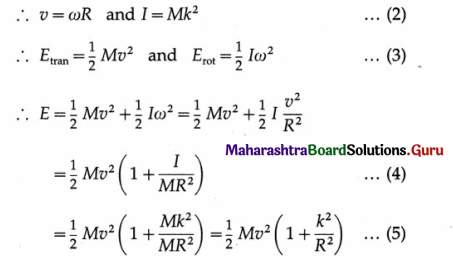Question 11.
A rigid object is rolling down an inclined plane. Derive expressions for the acceleration along the track and the speed after falling through a certain vertical distance.
Consider a circularly symmetric rigid body, like a sphere or a wheel or a disc, rolling with friction down a plane inclined at an angle 9 to the horizontal. If the frictional force on the body is large enough, the body rolls without slipping.
Let M and R be the mass and radius of the body. Let I be the moment of inertia of the body for rotation about an axis through its centre. Let the body start from rest at the top of the incline at aheight h. Let v be the translational speed of the centre of mass at the bottom of the incline. Then, its kinetic energy at the bottom of the incline is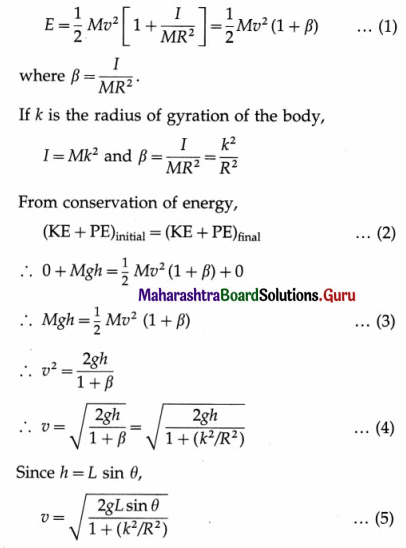Let a be the acceleration of the centre of mass of the body along the inclined plane. Since the body starts from rest,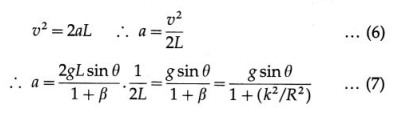Starting from rest, if t is the time taken to travel the distance L,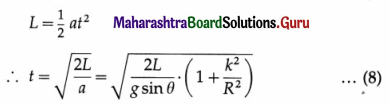[Note : For rolling without slipping, the contact point of the rigid body is instantaneously at rest relative to the surface of the inclined plane. Hence, the force of friction is static rather than kinetic, and does no work on the body. Thus, the force of static friction causes no decrease in the mechanical energy of the body and we can use the principle of conservation of energy.]

Question 12.
Somehow, an ant is stuck to the rim of a bicycle wheel of diameter 1 m. While the bicycle is on a central stand, the wheel
is set into rotation and it attains the frequency of 2 rev/s in 10 seconds, with uniform angular acceleration. Calculate
(i) Number of revolutions completed by the ant in these 10 seconds.
(ii) Time taken by it for first complete revolution and the last complete revolution.
[Ans:10 rev., tfirst = $$\sqrt{10}$$s, tlast = 0.5132s]
Data : r = 0.5 m, ω0 = 0, ω = 2 rps, t = 10 s

(i) Angular acceleration (α) being constant, the average angular speed,
ωav = $$\frac{\omega_{\mathrm{o}}+\omega}{2}$$ = $$\frac{0+2}{2}$$ = 1 rps
∴ The angular displacement of the wheel in time t,
θ = ωav ∙ t = 1 × 10 = 10 revolutions

(ii)The time for the last, i.e., the 10th, revolution is t1 – t2 = 10 – 9.486 = 0.514 s

Question 13.
Coefficient of static friction between a coin and a gramophone disc is 0.5. Radius of the disc is 8 cm. Initially the
centre of the coin is 2 cm away from the centre of the disc. At what minimum frequency will it start slipping from there? By what factor will the answer change if the coin is almost at the rim?
(use g = π2 m/s2)
[Ans: 2.5 rev/s, n2 = $$\frac{1}{2}$$n1]
Data : µs = 0.5, r1 = π cm = π × 10-2 m, r2 = 8 cm = 8 × 10-2 m, g = π2 m/s2
To revolve with the disc without slipping, the necessary centripetal force must be less than or equal to the limiting force of static friction.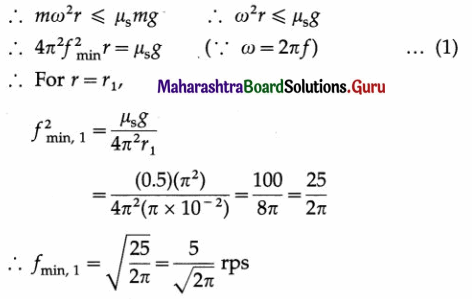The coin will start slipping when the frequency is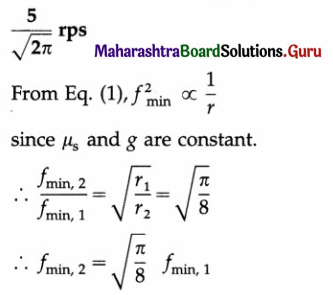The minimum frequency in the second case will be $$\sqrt{\frac{\pi}{8}}$$ times that in the first case.
[ Note The answers given in the textbook are for r1 = 2 cm.]

Question 14.
Part of a racing track is to be designed for a radius of curvature of 72 m. We are not recommending the vehicles to drive faster than 216 kmph. With what angle should the road be tilted? At what height will its outer edge be, with respect to the inner edge if the track is 10 m wide?
[Ans: θ = tan-1 (5) = 78.69°, h = 9.8 m]
Data : r = 72 m, v0 = 216 km/h, = 216 × $$\frac{5}{18}$$
= 60 m/s, w = 10 m, g = 10 m/s2
tan θ = $$\frac{v_{\mathrm{o}}^{2}}{r g}$$ = $$\frac{(60)^{2}}{72 \times 10}$$ = $$\frac{3600}{720}$$ = 5
∴ θ = tan-1 5 = 78°4′
This is the required angle of banking.
sin θ = $$\frac{h}{w}$$
∴ h = w sin θ = (10) sin 78°4′ = 10 × 0.9805
= 9.805 m
This gives the height of the outer edge of the track relative to the inner edge.

Question 15.
The road in the example 14 above is constructed as per the requirements. The coefficient of static friction between the tyres of a vehicle on this road is 0.8, will there be any lower speed limit? By how much can the upper speed limit exceed in this case?
[Ans: vmin ≅ 88 kmph, no upper limit as the road is banked for θ > 45°]
Data : r = 72 m, θ = 78 °4′, µs = 0.8, g = 10 m/s2 tan θ = tan 78°4′ = 5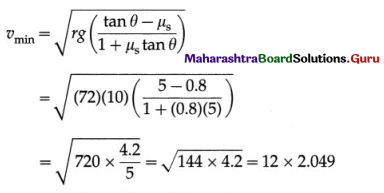= 24.588 m/s = 88.52 km/h
This will be the lower limit or minimum speed on this track.
Since the track is heavily banked, θ > 45 °, there is no upper limit or maximum speed on this track.

Question 16.
During a stunt, a cyclist (considered to be a particle) is undertaking horizontal circles inside a cylindrical well of radius 6.05 m. If the necessary friction coefficient is 0.5, how much minimum speed should the stunt artist maintain? Mass of the artist is 50 kg. If she/he increases the speed by 20%, how much will the force of friction be?
[Ans: vmin = 11 m/s, fs = mg = 500 N]
Data : r = 6.05 m, µs = 0.5, g = 10 m/s2, m = 50 kg, ∆v = 20%This is the required minimum speed. So long as the cyclist is not sliding, at every instant, the force of static friction is fs = mg = (50)(10) = 500 N

Question 17.
A pendulum consisting of a massless string of length 20 cm and a tiny bob of mass 100 g is set up as a conical pendulum. Its bob now performs 75 rpm. Calculate kinetic energy and increase in the gravitational potential energy of the bob. (Use π 2 = 10 )
[Ans: cos θ = 0.8, K.E. = 0.45 J, ∆(P E.) = 0.04 J]
Data : L = 0.2 m, m = 0.1 kg, n = $$\frac{75}{60}$$ = $$\frac{5}{4}$$ rps,
g = 10 m/s2, π2 = 10,The increase in gravitational PE,
∆PE = mg(L – h)
= (0.1) (10) (0.2 – 0.16)
= 0.04 JQuestion 18.
A motorcyclist (as a particle) is undergoing vertical circles inside a sphere of death. The speed of the motorcycle varies between 6 m/s and 10 m/s. Calculate diameter of the sphere of death. What are the minimum values are possible for these two speeds?
[Ans: Diameter = 3.2 m, (v1)min = 4 m/s, (v2)min = 4 $$\sqrt{5}$$/m s ]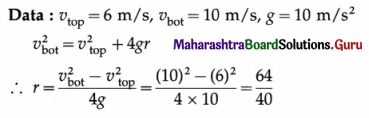= 1.6 m
The diameter of the sphere of death = 3.2 m.The required minimum values of the speeds are 4 m/s and 4$$\sqrt{5}$$ m/s.

Question 19.
A metallic ring of mass 1 kg has moment of inertia 1 kg m2 when rotating about one of its diameters. It is molten and remoulded into a thin uniform disc of the same radius. How much will its moment of inertia be, when rotated about its own axis.
[Ans: 1 kg m2]
The MI of the thin ring about its diameter,
Iring = $$\frac{1}{2}$$MR2 = 1 kg.m2
Since the ring is melted and recast into a thin disc of same radius R, the mass of the disc equals the mass of the ring = M.
The MI of the thin disc about its own axis (i.e., transverse symmetry axis) is
Idisc = $$\frac{1}{2}$$MR2 = Iring
∴ Idisc = 1 kg.m2

Question 20.
A big dumb-bell is prepared by using a uniform rod of mass 60 g and length 20 cm. Two identical solid thermocol spheres of mass 25 g and radius 10 cm each are at the two ends of the rod. Calculate moment of inertia of the dumbbell when rotated about an axis passing through its centre and perpendicular to the length.
[Ans: 24000 g cm-2]
Data : Msph = 50 g, Rsph = 10 cm, Mrod = 60 g, Lrod = 20 cm
The MI of a solid sphere about its diameter is
Isph,CM = $$\frac{2}{5}$$MsphRsph
The distance of the rotation axis (transverse symmetry axis of the dumbbell) from the centre of sphere, h = 30 cm.
The MI of a solid sphere about the rotation axis, Isph = Isph, CM + Msphh2
For the rod, the rotation axis is its transverse symmetry axis through CM.
Irod = $$\frac{1}{12}$$ MrodL2rod
Since there are two solid spheres, the MI of the dumbbell about the rotation axis is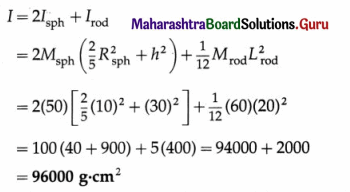Question 21.
A flywheel used to prepare earthenware pots is set into rotation at 100 rpm. It is in the form of a disc of mass 10 kg and
radius 0.4 m. A lump of clay (to be taken equivalent to a particle) of mass 1.6 kg falls on it and adheres to it at a certain
distance x from the centre. Calculate x if the wheel now rotates at 80 rpm.
[Ans: x = $$\frac{1}{\sqrt{8}}$$m = 0.35 m]
Data : f1 = 60 rpm = 60/60 rot/s = 1 rot/s,
f2 = 30 rpm = 30/60 rot/s = $$\frac{1}{2}$$ rot/s, ∆E = — 100 J

(i)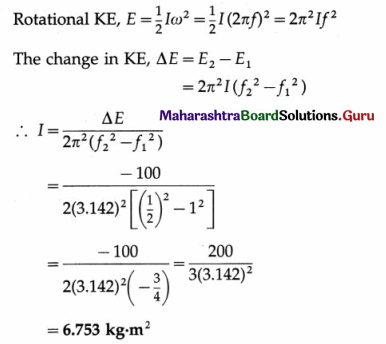This gives the MI of the flywheel about the given axis.

(ii) Angular momentum, L = Iω = I(2πf) = 2πIf
The change in angular momentum, ∆L
= L2 – L1 = 2πI(f2 – f1)
= 2 × 3.142 × 6.753($$\frac{1}{2}$$ – 1)
= -3.142 × 6.753 = -21.22 kg.m2/s

Question 22.
Starting from rest, an object rolls down along an incline that rises by 3 units in every 5 units (along it). The object gains a speed of $$\sqrt{10}$$ m/s as it travels a distance of $$\frac{5}{3}$$m along the incline. What can be the possible shape/s of the object?
[Ans: $$\frac{K^{2}}{R^{2}}$$ = 1. Thus, a ring or a hollow cylinder]
Data : sin θ = $$\frac{3}{5}$$, u = 0, v = $$\sqrt{10}$$ m/s, L = $$\frac{5}{3}$$m, g = 10 m/s2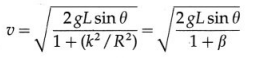Therefore, the body rolling down is either a ring or a cylindrical shell.

12th Physics Digest Chapter 1 Rotational Dynamics Intext Questions and Answers

Activity (Textbook Page No. 3)

Question 1.
Attach a body of suitable mass to a spring balance so that it stretches by about half its capacity. Now whirl the spring balance so that the body performs a horizontal circular motion. You will notice that the balance now reads more for the same body. Can you explain this ?
Due to outward centrifugal force.

Use your brain power (Textbook Page No. 4)

Question 1.
Obtain the condition for not toppling (rollover) for a four-wheeler. On what factors does it depend and how?
Consider a car of mass m taking a turn of radius r along a level road. As seen from an inertial frame of reference, the forces acting on the car are :

1. the lateral limiting force of static friction $$\overrightarrow{f_{\mathrm{s}}}$$ on the wheels-acting along the axis of the wheels and towards the centre of the circular path- which provides the necessary centripetal force,
2. the weight $$m \vec{g}$$ acting vertically downwards at the centre of gravity (C.G.)
3. the normal reaction $$\vec{N}$$ of the road on the wheels, acting vertically upwards effectively at the C.G. Since maximum centripetal force = limiting force of static friction,
mar = $$\frac{m v^{2}}{r}$$ = fs…. (1)

In a simplified rigid-body vehicle model, we consider only two parameters-the height h of the C.G. above the ground and the average distance b between the left and right wheels called the track width.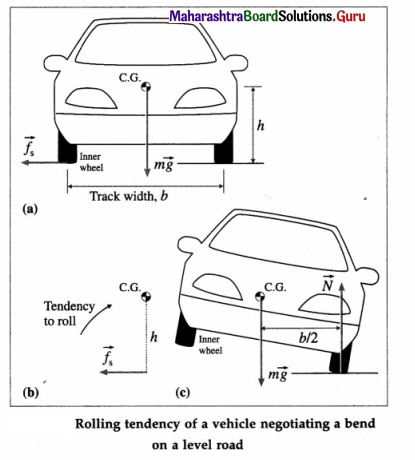The friction force $$\overrightarrow{f_{s}}$$ on the wheels produces a torque $$\tau_{\mathrm{t}}$$ that tends to overturn/rollover the car about the outer wheel. Rotation about the front-to-back axis is called roll.
$$\tau_{\mathrm{t}}$$ = fs.h = $$\left(\frac{m v^{2}}{r}\right)$$h … (2)

When the inner wheel just gets lifted above the ground, the normal reaction $$\vec{N}$$ of the road acts on the outer wheels but the weight continues to act at the C.G. Then, the couple formed by the normal reaction and the weight produces a opposite torque $$\tau_{\mathrm{r}}$$ which tends to restore the car back on all four wheels
$$\tau_{\mathrm{r}}$$ = mg.$$\frac{b}{2}$$ …. (3)
The car does not topple as long as the restoring torque $$\tau_{\mathrm{r}}$$ counterbalances the toppling torque $$\tau_{\mathrm{t}}$$. Thus, to avoid the risk of rollover, the maximum speed that the car can have is given by
$$\left(\frac{m v^{2}}{r}\right)$$h = mg.$$\frac{b}{2}$$ ∴ vmax = $$\sqrt{\frac{r b g}{2 h}}$$ … (4)

Thus, vehicle tends to roll when the radial acceleration reaches a point where inner wheels of the four-wheeler are lifted off of the ground and the vehicle is rotated outward. A rollover occurs when the gravitational force $$m \vec{g}$$ passes through the pivot point of the outer wheels, i.e., the C.G. is above the line of contact of the outer wheels. Equation (3) shows that this maximum speed is high for a car with larger track width and lower centre of gravity.

There will be rollover (before skidding) if $$\tau_{t}$$ ≥ $$\tau_{\mathrm{r}}$$, that is if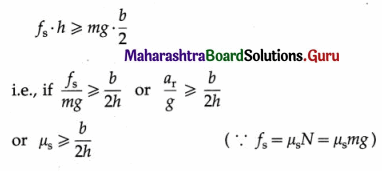The vehicle parameter ratio, $$\frac{b}{2 h}$$, is called the static stability factor (SSF). Thus, the risk of a rollover is low if SSF ≤ µs. A vehicle will most likely skid out rather than roll if µs is too low, as on a wet or icy road.

Question 2.
Think about the normal reactions. Where are those and how much are those?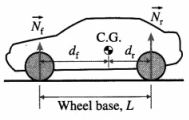In a simplified vehicle model, we assume the normal reactions to act equally on all the four wheels, i.e., mg/4 on each wheel. However, the C.G. is not at the geometric centre of a vehicle and the wheelbase (i.e., the distance L between its front and rear wheels) affects the weight distribution of the vehicle. When a vehicle is not accelerating, the normal reactions on each pair of front and rear wheels are, respectively,
Nf = $$\frac{d_{\mathrm{r}}}{L}$$mg and Nr = $$\frac{d_{\mathrm{f}}}{L}$$ mg
where dr and df are the distances of the rear and front axles from the C.G. [When a vehicle accelerates, additional torque acts on the axles and the normal reactions on the wheels change. So, as is common experience, a car pitches back (i.e., rear sinks and front rises) when it accelerates, and a car pitches ahead (i.e., front noses down). Rotation about the lateral axis is called pitch.]

Question 3.
What is the recommendations on loading a vehicle for not toppling easily?
Overloading (or improper load distribution) or any load placed on the roof raises a vehicle’s centre of gravity, and increases the vehicle’s likelihood of rolling over. A roof rack should be fitted by considering weight limits.

Road accidents involving rollovers show that vehicles with higher h (such as SUVs, pickup vans and trucks) topple more easily than cars. Untripped rollovers normally occur when a top-heavy vehicle attempts to perform a panic manoeuver that it physically cannot handle.

Question 4.
If a vehicle topples while turning, which wheels leave the contact with the road? Why?
Inner wheels.
Consider a car of mass m taking a turn of radius r along a level road. As seen from an inertial frame of reference, the forces acting on the car are :

1. the lateral limiting force of static friction $$\overrightarrow{f_{\mathrm{s}}}$$ on the wheels-acting along the axis of the wheels and towards the centre of the circular path- which provides the necessary centripetal force,
2. the weight $$m \vec{g}$$ acting vertically downwards at the centre of gravity (C.G.)
3. the normal reaction $$\vec{N}$$ of the road on the wheels, acting vertically upwards effectively at the C.G. Since maximum centripetal force = limiting force of static friction,
mar = $$\frac{m v^{2}}{r}$$ = fs…. (1)

In a simplified rigid-body vehicle model, we consider only two parameters-the height h of the C.G. above the ground and the average distance b between the left and right wheels called the track width.The friction force $$\overrightarrow{f_{s}}$$ on the wheels produces a torque $$\tau_{\mathrm{t}}$$ that tends to overturn/rollover the car about the outer wheel. Rotation about the front-to-back axis is called roll.
$$\tau_{\mathrm{t}}$$ = fs.h = $$\left(\frac{m v^{2}}{r}\right)$$h … (2)

When the inner wheel just gets lifted above the ground, the normal reaction $$\vec{N}$$ of the road acts on the outer wheels but the weight continues to act at the C.G. Then, the couple formed by the normal reaction and the weight produces a opposite torque $$\tau_{\mathrm{r}}$$ which tends to restore the car back on all four wheels
$$\tau_{\mathrm{r}}$$ = mg.$$\frac{b}{2}$$ …. (3)
The car does not topple as long as the restoring torque $$\tau_{\mathrm{r}}$$ counterbalances the toppling torque $$\tau_{\mathrm{t}}$$. Thus, to avoid the risk of rollover, the maximum speed that the car can have is given by
$$\left(\frac{m v^{2}}{r}\right)$$h = mg.$$\frac{b}{2}$$ ∴ vmax = $$\sqrt{\frac{r b g}{2 h}}$$ … (4)

Thus, vehicle tends to roll when the radial acceleration reaches a point where inner wheels of the four-wheeler are lifted off of the ground and the vehicle is rotated outward. A rollover occurs when the gravitational force $$m \vec{g}$$ passes through the pivot point of the outer wheels, i.e., the C.G. is above the line of contact of the outer wheels. Equation (3) shows that this maximum speed is high for a car with larger track width and lower centre of gravity.

Question 5.
How does [tendency to] toppling affect the tyres?
While turning, shear stress acts on the tyre-road contact area. Due to this, the treads and side wall of a tyre deform. Apart from less control, this contributes to increased and uneven wear of the shoulder of the tyres.

Each wheel is placed under a small inward angle (called camber) in the vertical plane. Under severe lateral acceleration, when the car rolls, the camber angle ensures the complete contact area is in contact with the road and the wheels are now in vertical position. This improves the cornering behavior of the car. Improperly inflated and worn tyres can be especially dangerous because they inhibit the ability to maintain vehicle control. Worn tires may cause the vehicle to slide
sideways on wet or slippery pavement, sliding the vehicle off the road and increasing its risk of rolling over.Question 6.
What is the recommendation for this?
Because of uneven wear of the tyre shoulders, tyres should be rotated every 10000 km-12000 km. To avoid skidding, rollover and tyre-wear, the force of friction should not be relied upon to provide the necessary centripetal force during cornering. Instead, the road surface at a bend should be banked, i.e., tilted inward.

A car while taking a turn performs circular motion. If the road is level (or horizontal road), the necessary centripetal force is the force of static friction between the car tyres and the road surface. The friction depends upon the nature of the surfaces in contact and the presence of oil and water on the road. If the friction is inadequate, a speeding car may skid off the road. Since the friction changes with circumstances, it cannot be relied upon to provide the necessary centripetal force. Moreover, friction results in fast wear and tear of the tyres.

To avoid the risk of skidding as well as to reduce the wear and tear of the car tyres, the road surface at a bend is tilted inward, i.e., the outer side of the road is raised above its inner side. This is called banking of road. On a banked road, the resultant of the normal reaction and the gravitational force can act as the necessary centripetal force. Thus, every car can be safely driven on such a banked curve at certain optimum speed, without depending on friction. Hence, a road should be properly banked at a bend.

The angle of banking is the angle of inclination of the surface of a banked road at a bend with the horizontal.

When a two-wheeler takes a turn along an unbanked road, the force of friction provides the centripetal force. The two-wheeler leans inward to counteract a torque that tends to topple it outward. Firstly, friction cannot be relied upon to provide the necessary centripetal force on all road conditions. Secondly, the friction results in wear and tear of the tyres. On a banked road at a turn, any vehicle can negotiate the turn without depending on friction and without straining the tyres.

Question 7.
Determine the angle to be made with the vertical by a two-wheeler while turning on a horizontal track?

When a bicyclist takes a turn along an unbanked road, the force of friction $$\vec{f}_{\mathrm{s}}$$ provides the centripetal force; the normal reaction of the road $$\vec{N}$$ is vertically up. If the bicyclist does not lean inward, there will be an unbalanced outward torque about the centre of gravity, fs.h, due to the friction force that will topple the bicyclist outward. The bicyclist must lean inward to counteract this torque (and not to generate a centripetal force) such that the opposite inward torque of the couple formed by $$\vec{N}$$ and the weight $$\vec{g}$$, mg.a = fs.h1Since the force of friction provides the centripetal force,
fs = $$\frac{m v^{2}}{r}$$
If the cyclist leans from the vertical by an angle 9, the angle between $$\vec{N}$$ and $$\vec{F}$$ in above figure.Hence, the cyclist must lean by an angle
θ = tan-1$$\left(\frac{v^{2}}{g r}\right)$$

When a car takes a turn along a level road, apart from the risk of skidding off outward, it also has a tendency to roll outward due to an outward torque about the centre of gravity due to the friction force. But a car is an extended object with four wheels. So, when the inner wheels just get lifted above the ground, it can be counterbalanced by a restoring torque of the couple formed by the normal reaction (on the outer wheels) and the weight.

Question 8.
We have mentioned about ‘static friction’ between road and tyres. Why is it static friction? What about kinetic friction between road and tyres?
When a car takes a turn on a level road, the point of contact of the wheel with the surface is instantaneously stationary if there is no slipping. Hence, the lateral force on the car is the limiting force of static friction between the tyres and road. Lateral forces allow the car to turn. As long as the wheels are rolling, there is lateral force of static friction and longitudinal force of rolling friction. Longtitudinal forces, which act in the direction of motion of the car body (or in the exact opposite direction), control the acceleration or deceleration of the car and therefore the speed of the car. These are the wheel force, rolling friction, braking force and air drag. If the car skids, the friction force is kinetic friction; more importantly, the direction of the friction force then changes abruptly from lateral to that opposite the velocity of skidding and not towards the centre of the curve, so that the car cannot continue in its curved path.

Question 9.
What do you do if your vehicle is trapped on a slippery or sandy road? What is the physics involved?
Driving on a country road should be attempted only with a four-wheel drive. However, if you do get stuck in deep sand or mud, avoid unnecessary panic and temptation to drive your way out of the mud or sand because excessive spinning of your tyres will most likely just dig you into a deeper hole. Momentum is the key to getting unstuck from sand or mud. One method is the rocking method-rocking your car backwards and forwards to gain momentum. Your best option is usually to gain traction and momentum by wedging a car mat (or sticks, leaves, gravel or rocks) in front and under your drive wheels. Once you start moving, keep the momentum going until you are on more solid terrain.

Use your brain power (Textbook Page No. 6)

Question 1.
As a civil engineer, you are to construct a curved road in a ghat. In order to calculate the banking angle 0, you need to decide the speed limit. How will you decide the values of speed and radius of curvature at the bend ?
For Indian roads, Indian Road Congress (IRC), [IRC-73-1980, Table 2, p.4], specifies the design speed depending on the classification of roads (such as national and state highways, district roads and village roads) and terrain. It is the basic design parameter which determines further geometric design features. For the radius of curvature at a bend, IRC [ibid., Table 16, p.24] specifies the absolute minimum values based on the minimum design speed. However, on new roads, curves should be designed to have the largest practicable radius, generally more than the minimum values specified, to allow for ‘sight distance’ and ‘driver comfort’. To consider the motorist driving within the innermost travel lane, the radius used to design horizontal curves should be measured to the inside edge of the innermost travel lane, particularly for wide road-ways with sharp horizontal curvature.

A civil engineer refers to banking as superelevation e;e = tan θ. IRC fixes emax = 0.07 for a non-urban road and the coefficient of lateral static friction, µ = 0.15, the friction between the vehicle tyres and the road being incredibly variable. Ignoring the product eµ, from Eq. (6)
e + µ = $$\frac{v^{2}}{g r}$$ (where both v and r are in SI units)
= $$\frac{V^{2}}{127 r}$$(where V is in km / h and r is in metre) …. (1)
The sequence of design usually goes like this :

1. Knowing the design speed V and radius r, calculate the superelevation for 75% of design speed ignormg friction : e = $$\frac{(0.75 \mathrm{~V})^{2}}{127 r}$$ = $$\frac{V^{2}}{225 r}$$
2. If e < 0.07, consider this calculated value of e in subsequent calculations. If e > 0.07, then take e = emax = 0.07.
3. Use Eq. (1) above to check the value of µ for emax = 0.07 at the full value of the design speed V : µ = $$\frac{V^{2}}{127 r}$$ – 0.07
If µ < 0.15, then e = 0.07 is safe. Otherwise, calculate the allowable speed Va as in step 4.
4. $$\frac{V_{\mathrm{a}}^{2}}{127 r}$$ = e + p = 0.07 + 0.15
If Va > V, then the design speed V is adequate.
If Va < V, then speed is limited to Va with appropriate warning sign.

Use your brain power (Textbook Page No. 7)

Question 1.
If friction is zero, can a vehicle move on the road? Why are we not considering the friction in deriving the expression for the banking angle?
Friction is necessary for any form of locomotion. Without friction, a vehicle cannot move. The banking angle for a road at a bend is calculated for optimum speed at which every vehicle can negotiate the bend without depending on friction to provide the necessary lateral centripetal force.

Question 2.
When a car takes a turn on a level road, the point of contact of the wheel with the surface is instantaneously stationary if there is no slipping. Hence, the lateral force on the car is the limiting force of static friction between the tyres and road. Lateral forces allow the car to turn. As long as the wheels are rolling, there is lateral force of static friction and longitudinal force of rolling friction. Longtitudinal forces, which act in the direction of motion of the car body (or in the exact opposite direction), control the acceleration or deceleration of the car and therefore the speed of the car. These are the wheel force, rolling friction, braking force and air drag. If the car skids, the friction force is kinetic friction; more importantly, the direction of the friction force then changes abruptly from lateral to that opposite the velocity of skidding and not towards the centre of the curve, so that the car cannot continue in its curved path.

Use your brain power (Textbook Page No. 12)

Question 1.
What is expected to happen if one travels fast over a speed breaker? Why?
The maximum speed with which a car can travel over a road surface, which is in the form of a convex arc of radius r, is $$\sqrt{r g}$$ where g is the acceleration due to gravity. For a speed breaker, r is very small (of the order of 1 m). Hence, one must slow down considerably while going over a speed breaker. Otherwise, the car will lose contact with the road and land with a thud.

Question 2.
How does the normal force on a concave suspension bridge change when a vehicle is travelling on it with a constant speed ?
At the lowest point, N-mg provides the centripetal force. Therefore, N-mg = $$\frac{m v^{2}}{r}$$, so that N = m(g + $$\frac{v^{2}}{r}$$).